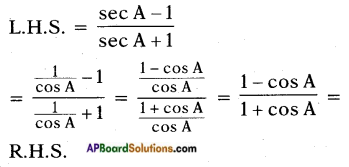# AP SSC 10th Class Maths Solutions Chapter 11 Trigonometry Optional Exercise

## AP State Syllabus SSC 10th Class Maths Solutions 11th Lesson Trigonometry Optional Exercise

AP State Board Syllabus AP SSC 10th Class Maths Textbook Solutions Chapter 11 Trigonometry Optional Exercise Textbook Questions and Answers.

### 10th Class Maths 11th Lesson Trigonometry Optional Exercise Textbook Questions and Answers

Question 1.
Prove that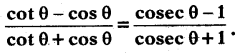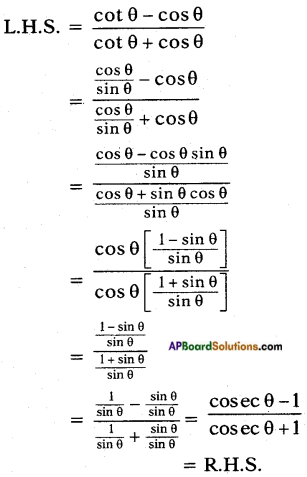Hence Proved.Question 2.
Prove that $$\frac{\sin \theta-\cos \theta+1}{\sin \theta+\cos \theta-1}$$ = $$\frac{1}{\sec \theta-\tan \theta}$$ using the identity sec2 θ = 1 + tan2 θ.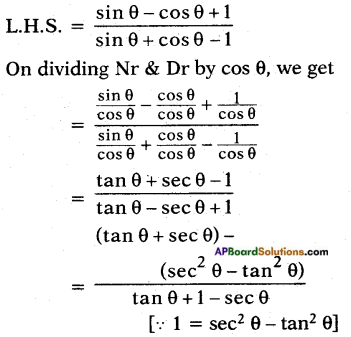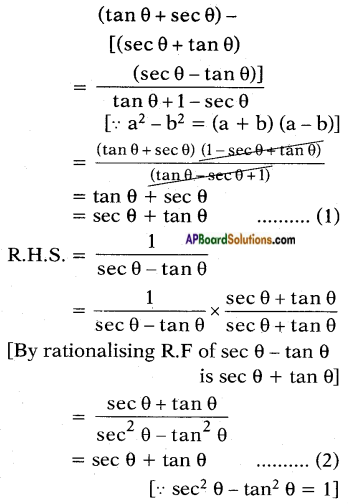From (1) and (2), we get L.H.S. = R.H.S.
Hence Proved.Question 3.
Prove that (cosec A – sin A) (sec A – cos A) = $$\frac{1}{\tan A+\cot A}$$
L.H.S. = (cosec A – sin A) (sec A – cos A)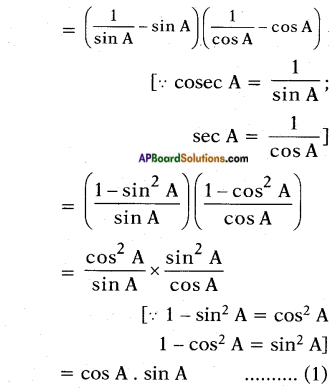Now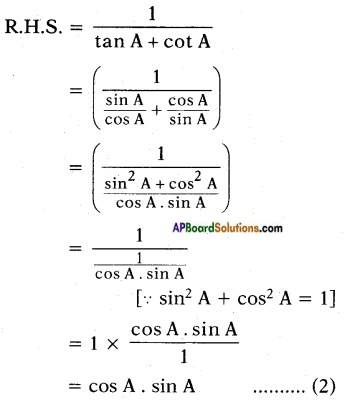From (1) and (2), we get
L.H.S. = R.H.S.
∴ (cosec A – sin A) (sec A – cos A) = $$\frac{1}{\tan A+\cot A}$$Question 4.
Prove that $$\frac{1+\sec A}{\sec A}$$ = $$\frac{\sin ^{2} A}{1-\cos A}$$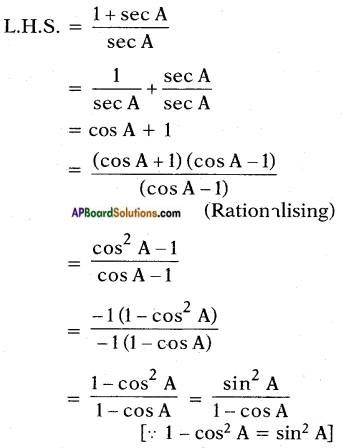Question 5.
Prove that $$\left(\frac{1+\tan ^{2} A}{1+\cot ^{2} A}\right)$$ = $$\left(\frac{1+\tan A}{1+\cot A}\right)^{2}$$ = tan2 A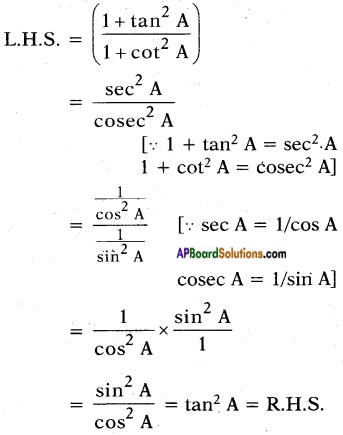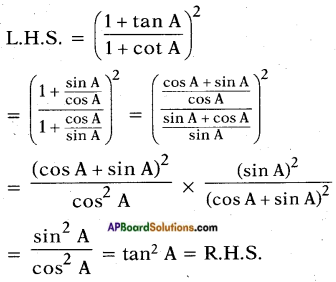Prove that $$\left(\frac{\sec A-1}{\sec A+1}\right)$$ = $$\left(\frac{1-\cos A}{1+\cos A}\right)$$